## Secret Sharing

Lecturer: Lidong Zhou

Lecture notes by Michael Frei

### Secret Sharing

Suppose you and your friend accidentally discovered a map that you believe would lead you to an island full of treasure. You and your friend are very excited and would like to go home and get ready for the exciting journey to the great fortune. Now who is going to keep the map? Suppose you and your so-called friend do not really trust each other and are afraid that, if the other one has the map, he/she might just go alone and take everything. Now we need a scheme that could make sure that the map is shared in a way so that no one would be left out in this trip. What would you suggest?

An easy way to solve this problem is to split the map into two pieces and make sure that both pieces are needed in order to find the island. Now we give one piece to each. You can happily go home and be assured that your friend has to go with you in order to find the island. This illustrates the basic concept of secret sharing.

#### Generalization

Let's generalize the idea. Given a secret `s`, we would like `n` parties to share the secret so that the following properties hold:
1. All n parties can get together and recover `s`.
2. Less than n parties cannot recover `s`.

In the map example, `s` is the map, while you and your friends are two parties that share the secret. In general, to achieve such a sharing, we split the secret into `n` pieces `s1, s2, . . ., sn ` and give one piece to each party. Each piece here is called a share. This is actually a special case of secret sharing and is called `secret splitting` in some literature.

We know that every piece of information is stored as a bit string or a number on a computer. So, if we know how to share a secret bit string or a secret number, then we know how to share any information on a computer. For example, assume that your salary is stored as a number 12345678. Now you want to split your salary into two shares for two parties. You want to make sure that no party by itself knows your salary. On the other hand, two parties can get together and recover your salary.

We can apply the same approach as we did to the map. We can split the digits into two sets and give one set to each party as a share. For example, can give the first 4 digits to party 1 and the other 4 to party 2. It is easy to verify that this scheme satisfies the two properties listed.

However, there is a problem with this scheme. Suppose I am the first party who gets the most significant 4 digits of your salary. It is true that I don't know exactly how much your salary is, but I have a pretty good idea about the range of your salary (>= 12340000), because I have the 4 most significant digits. Such partial information may be unacceptable for you.

### Partial Information Disclosure

The problem illustrated here is really a partial information disclosure problem. A share may not contain all the information about a secret, but could disclose partial information. In certain cases, such partial information disclosure could be fatal.

Suppose two parties are going to share a password. Here a password consists of 8 characters, with each selected from a set of 100 possible characters. If we split the password into two shares and each share has 4 characters of the password, then each share effectively gives out the secret under a brute-force attack.

A brute-force attack tries every possible combination of the the password in order to find the right password. So now let's do some calculations. There are 100 possible characters to choose from for each of the 8 characters, so there are 1008 possible passwords. If it takes 1 microsecond to generate and check one password, then it takes 1008 * 10-6 seconds ~ 300 years to try every possible password. Of course, on average, you only need to try half of the possible passwords, but still it takes more than 100 years. What if the adversary has one share, which means that the adversary knows 4 characters? Now there are only 1004 possible passwords to check against. It would only take 1004 * 10-6 = 100 sec to find the password. Now you see that a naive way of splitting a secret could cause partial information disclosure, which might be undesirable in certain cases and fatal in others.

We would like to solve the partial information disclosure problem: we strengthen property 2 to stipulate that no information about the secret will be disclosed from less than n shares. It may seem counter-intuitive that the shares generated from a secret can contain no information about the secret, but you will be surprised how easily one can design a scheme that ensures these two new properties.

Let's illustrate the scheme with a simple example. Suppose two parties are going to share a secret bit string 1011. The two shares are generated as follows (see Figure 1 below):

1. To generate the first bit of the two shares, we flip a coin. If the result of the coin flipping is a head, then the first bit of the first share is 0; if the result of the coin flipping is a tail, then the first bit of the first share is a 1.
2. Now generate the first bit of the second share. If the result of the previous coin flipping was a head, then we copy the first bit of the secret. Else, if result of the previous coin flipping was a tail, then we flip the first bit of the secret and use that. (Note: in fact, what we have simply done is to make the first bit of the second share equal to the XOR of the first bit of the first share and the first bit of the secret.)
3. We repeat this random process for each bit of the secret.

Suppose for our example where the secret bit string is 1011, we flip the coin 4 times and get the sequence head, tail, tail, and head. Then the bits of our first share would simply be 0110. As a result, the bits of the second share would be 1101 (the XOR of 0110 and 1011). (see Figure 1 below)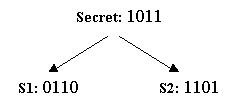Now we need to show that this scheme satisfies the two properties mentioned earlier. First, it is easy to see that the secret can be reconstructed from the two shares using bit-wise XOR. Second, we also need to show that the secret cannot be recovered with less than two shares. We know that the first share is generated through coin flipping. Obviously, it is random and has nothing to do with the secret. As for the second share, it is the result of a random transformation, based on coin flipping, of the secret. It should also be random.

If this argument is not convincing enough, then let's look at another argument. Suppose you have the first share (see Figure 2 below). Since you can't see the first share or the secret, you cannot distinguish the two cases. Therefore you cannot decide which secret you're sharing, and you can't figure out even a single bit of the secret.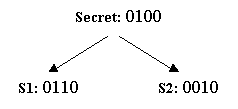If you had the second share (see Figure 3 below), the situation is the same. Again, you cannot learn any bit of the secret from your share alone.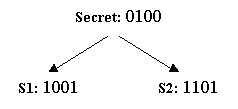The precise meaning of "no information disclosure" can be formalized, but we don't want to get into the formal details. Hopefully by now you are convinced that the scheme works. We can easily generalize the scheme for cases where there are n parties: we generate n-1 random bit strings (with the same number of bits as the secret) as the first n-1 shares. The last share is the result of bitwise XORing the secret with all the other n-1 shares.

#### Modifying Disclosure Conditions

Now we have this nice secret splitting scheme. But such a secret splitting scheme may not suffice in certain cases.

Imagine that you are asked to design a control mechanism for a nuclear missile launch. There is a control panel with a key board. You can enter a secret code through the keyboard. If the secret code is correct, then the missile gets launched. There are three generals who are in charge of a missile launch.

A simple solution would be to give the secret code to these three generals, but then it is possible for a lunatic general to start a war and destroy the planet. Hey, people make mistakes! We can see that we need some sort of secret sharing here. Let's use the secret splitting scheme we just developed. We will generate 3 shares from the secret code and give one share to each general.

Because of the second property of secret splitting, we know that no single general is able to launch the missile by himself, because each general has only one share and has no information about the secret code. On the other hand, what if one general is a spy from a hostile country? Well, we're not worried about him launching the missile by himself. But he can disable the missile launch capability by throwing away his share. Because the other two generals cannot authorize a missile launch without the third share, no missile can be launched, even if the country is under attak. Even if no general is a spy, what happens if one general is sick or is on vacation. Again, the country loses its capability to launch the missile.

The problem in the previous example is really the availability of the secret code. This is an essential issue in this example because the capability to launch a missile depends on the availability of the secret code. Assuming that it is unlikely that more than 1 general could be compromised or unavailable, we may postulate the following policies:

1. A missile can be launched with 2 or more generals
2. Less than 2 generals may not launch a missile.

#### (n,t) Secret Sharing

To generalize the properties, we get (n,t) secret sharing. Given a secret `s`, to be shared among n parties, that sharing should satisfy the following properties:

1. Availability: greater than or equal to t parties can recover `s`.
2. Confidentiality: less than t parties have no information about `s`.

In the missile launch example, we are in fact using a (3,2) secret sharing scheme. Also note that a secret splitting scheme is simply a special case of secret sharing where n is equal to t.

Let's see how we can design an (n,t) secret sharing scheme. To make the presentation easy to understand, let's start with the design of an (n,2) scheme.

Let's say we want to share a secret `s` among n parties. We use some basic geometry (see Figure 4 below). Select the point (0, s) on the Y axis that corresponds to the secret. Now, randomly draw a line that goes through this point. Pick n points on that line: ```(x1, y1), (x2, y2), ..., (xn, yn)```. Each point that is picked represents a share. We claim that these n shares constitute an (n,2) sharing of `s`. Now we need to show that this scheme satisfies both the availability and confidentiality properties.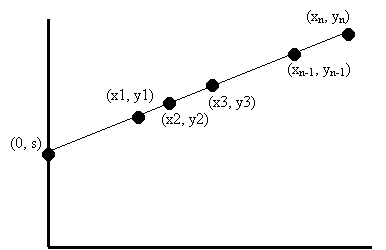To show availability, we need to prove that two parties can recover the secret. Two parties have two shares; that is two points. Given these two points, how can we recover the secret? Well, we know that two points determine a line, so we can figure out the line that goes through both points. Once we know the line, we know the intersection of the line with the y axis. Then, we get the secret. So, it only takes us two points (shares) to make the secret available.

What about confidentiality? We need to show that one share does not disclose any information about the secret. There are infinite possible lines that go through this point, and these lines intersect with the y-axis at different points, all of which yield different "secrets". In fact, given any possible secret, we can draw a line that goes through the secret and the given share. This means that with one point, no information about the secret is exposed.

Using the same idea, can we design an (n, 3) secret sharing scheme? Note that the key point in the (n,2) scheme is that a line is determined by two points, but not by 1. Now we need a curve that is determined by three points, but not 2. This curve happens to correspond to a quadratic function y = a2*x2 + a1 * x + a0. Again, we find the point on the y-axis that corresponds to the secret, then we randomly select a curve corresponding to a quadratic function that goes through the point. Finally, we select n points on that curve as n shares to n parties (see Figure 5 below). Using a similar proof as in the (n, 2) case, we can show that this is actually an (n, 3) scheme that satisfies both availability and confidentiality.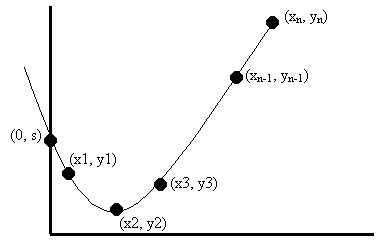To generalize the scheme even further, we have a construction of an (n, t) secret sharing scheme. Now we use the curve that corresponds to a (t-1) degree polynomial:

y = at-1 * xt-1 + at-2 * xt-2 + ... + a1 * x + a0

We randomly select a curve corresponding to such a polynomial that goes through the secret on the y-axis. And then we select n points on the curve. Using the same arguments, we can show that this scheme satisfies both availability and confidentiality properties. (Note: this scheme was invented by Shamir in 1979. The original scheme was defined on a finite field.)

### Online Secret Sharing

We have talked about how to design an (n, t) secret sharing scheme. Suppose now we want to maintain a secret on-line. We can store the secret on one server. However, if we do so, the secret would be disclosed if the server is compromised. If the server is corrupted, then the secret may be corrupted or lost.

If you have taken a Distributed Systems class, you know that we can use replication to improve the availability of a service and achieve fault tolerance. Naive replication does improve availability, but the service gets more vulnerable.

To solve these problems, we can split the secret into three shares using (3,2) secret sharing scheme and give one share to one server. Now, assuming that it is unlikely that two servers would be compromised, the secret will remain available and confidential. Even if an adversary steals a share from one server, the adversary does not know the secret because a (3, 2) secret sharing scheme is used -- a single share exposed no information about the secret. In the even that a share is lost because of the collapse of one server, we still have two shares to recover the secret.

However, there is another problem that we have not addressed: how does a compromised server recover? Traditionally, server recovery is performed by re-starting the server with a clean copy of the code and the up-to-date state. Such recovery is no longer sufficient if a secret share is stored on this server.

Let's look at what could happen over time. We have these three servers with three shares, a (3, 2) sharing of a secret s. In January, server 3 was attacked and share s3 is disclosed. We recovered the server immediately. In May, the same attacker successfully broke into server 2 and grabbed share s2 (see Figure 6 below). Now, this attacker has two shares and thus is able to recover the secret!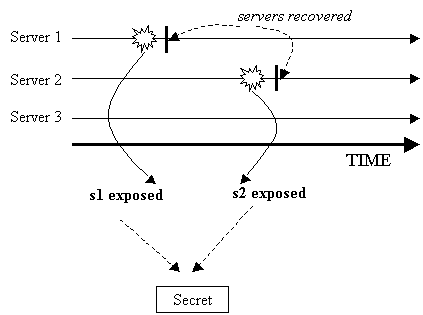The problem lies in the fact that the recovery of server 3 was not complete. One thing we should have done is to make any possibly exposed share obsolete. What do we mean by making a share "obsolete"? Well, share s3, by itself, does not contain any information about the secret. But it can be used together with share s1 or s2 to recover s. If shares s1 and s2 are deleted (or forgotten) by the other two servers, then s3 becomes useless (i.e., obsolete). The information on s3 is useless without s2 and s1.

We cannot simply ask servers 1 and 2 to delete their shares, because we still want the servers to maintain the secret. What we can do, on the other hand, is to have another (3, 2) sharing of the same secret s, say s1', s2', s3', to replace the old shares. If we have a trusted entity, then this resharing can be performed as follows. The servers first submit their shares to this trusted entity through secure links. The trusted entity can then recover the secret from these shares and generate a new set of shares (s1', s2', s3') for s using a (3, 2) sharing scheme. Note that the scheme to generate shares is random, so the new shares should be totally independent from the old ones. That is, knowing two points on two different lines gives no disclosure of the secret.

Unfortunately, we don't have the luxury of a trusted entity. In fact, such a trusted entity could become a vulnerability in the system; if the trusted entity is compromised when it performs re-sharing, then the secret could be disclosed. Therefore, we have to rely on the servers themselves to do re-sharing in a distributed manner. Again, no server can ever reconstruct the secret because otherwise the secret could be exposed if the server was compromised.

#### Proactive Secret Sharing

We need a scheme that allows servers to generate a new set of shares for the same secret from the old shares without reconstructing the secret. Such a scheme is called a proactive secret scheme (PSS). We have argued that PSS is needed for server recovery. But, in reality, break-ins to a server are very hard to detect, especially when the attacker simply steals certain secret information without modifying anything on the victim server. An attacker can cover his tracks when he exits. To strengthen the security of a replicated service, we can invoke our PSS periodically (at regular intervals). (see Figure below)Before the execution of the PSS, every server checks the integrity of its code and state, trying to remove any attackers that might exist in that server at that point in time. How would our PSS improve security through periodic executions? Well, with no PSS, using an (n, t) secret sharing scheme, a service can tolerate up to t-1 compromised servers during the entire lifetime of the service, because any more failures could lead to the exposure of the secret. With a PSS, we know that the PSS refreshes all the shares, so that old shares become useless. Now an adversary has to gather enough shares (at least t) between two executions of the PSS, which obviously makes the attackers job more difficult. The secret remains confidential if fewer than t servers could be compromised from the start of one PSS to the end of the next PSS.

To show how a proactive scheme can be achieved, let's study a simple example first. We first assume that an adversary can only break into a server and have access to information stored or collected by that server. The adversary cannot change the code of the server. Suppose we have a simple (2, 2) sharing scheme. To generate two shares for secret `s`, we randomly select s1 and s2, so that s1 + s2 = s. We want the two servers with shares s1 and s2 to change their shares to s1' and s2', so that these two shares remain an (2,2) sharing of the same secret s and these two shares are independent from the old shares (cannot be inferred from the old shares). The proactive secret sharing can be performed in the following steps:

1. Server 1 generates two subshares s11 and s12 from its share s1 using the same secret sharing scheme as the one used to generate s1 and s2 from s; that is, server 1 randomly selects two subshares s11 and s12, so that s1 = s11 + s12,. Server 2 does the same thing to s2: It randomly generates two subshares s21 and s22, so that s2 = s21 + s22.

2. Server 1 sends s12 to server 2 through a certain secure channel. Server 2 sends s21 to Server 1.

3. Server 1 has both s11 and s21 and can add them up to get a new share s1' = s11 + s21. Server 2, on the other hand, has both s12 and s22 and can generate a new share s2' = s12 + s22. Now we show that s1' and s2' constitute a (2,2) sharing. The sum of these two shares is the sum of all the four subshares, which is the sum of s1 and s2, which is s.

These two shares are independent from the old ones because these subshares are generated randomly. Also, no server knows the secret during the entire process. Server 1 generates s11 and s12 and learns s21 from server 2, but server 1 never knows s22 and thus does not know s2' or s. Server 2, on the other hand, never knows s11, and thus does not know s1' or s. (see Figure 8 below)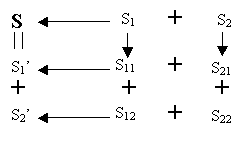#### Proactive (n, t) Secret Sharing

Before we describe the PSS scheme for (n, t) secret sharing, we first describe an interesting property of Shamir's scheme. This property is the basis for our proactive secret sharing scheme. We first simplify the (n, t) scheme we described earlier. We set the x-coordinates of the shares to x1=1, x2=2, ..., xn=n. Now the share si can simply be the y coordinate of a point (since the x-coordinates of these points are fixed and known).

Let's start with the (n, 2) scheme. We define an "add" operation on two lines as follows. For any x, the height of the point on the new line (with x the x coordinator being x) is the heights of the points on the two lines added together. Specifically, if the two initial lines intersect with the Y-axis at (0,s) and (0,s'), then the new line intersects with the Y-axis at (0, s+s'). In another view, the two lines can be represented as:

`f(x) = a1*x + s`
`h(x) = a2*x + s'`

The new line ` f'(x) = f(x) + h(x) = (a1 + a2)*x + (s + s')`. Each line can correspond to an (n, 2) sharing of a secret. From f(x), we get a sharing of ```s: (s1, s2, .., sn) ```, and from h(x), we get a sharing of `s': (s1', s2', .., sn')`. Now it is easy to see that `(s1 + s1', s2 + s2', .., sn + sn')` are the y-coordinates of the n points on the new line corresponding to `f'(x)`. These shares actually constitute an (n, 2) sharing of s+s'. As a special case, if s' = 0, then we get another sharing of the same secret s. This is the basis of our PSS for an (n, 2) secret sharing scheme.

A server can randomly select a function in the form of `h(x) = b1*x`. This server computes `h(i)` and sends `h(i)` to server i through a secure channel. Each server i computes its new share `f'(i) = f(i) + h(i).` Due to the property we just illustrated, `(f'(1), f'(2), ..., f'(n))` constitutes an (n, 2) sharing of s, just as `(f(1), f(2), ..., f(n))` does.

We can easily extend the idea to the (n, t) secret sharing case, thanks to the same addition property. For an (n, t) sharing, the function ```f(x) = at-1 * xt-1 + at-2 * xt-2 + ... + a1*x + s.```

A server selects a function ```h(x) = bt-1 * xt-1 + bt-2 * xt-2 + ... + b1*x.``` For every 1 <= i <= n, the server computers h(i) and sends h(i) to server i (through secure channel).

The new curve where the new shares are selected corresponds to function `f'(x) = f(x) + h(x)`. Server i then computes the new share `f'(i) = f(i) + h(i)`.

However, this extension has a severe security flaw. In cases where t > 2, it is not enough to let only one server select h(x): if the server that picks h(x) is compromised during PSS, then the adversary may know the relationship between new shares and old shares. More precisely, the knowledge of h(x) may enable the adversary to figure out si from si', even if si has been deleted. For example, consider a (5,3) sharing. Assume that server 1 (who selects h(x)) is compromised before PSS, and server 3 after. The adversary knows shares s1 (s1'), s2, and s3'. Moreover, the adversary can find out s2' from s2 (s2' = f'(2) = f(2) + h(2) = s2 + h(2)). Then, the adversary has s1', s2', and s3', and consequently, s, even though less than 3 servers are compromised from the start of a PSS to the end of the next PSS.

As a countermeasure, we ask (t-1) servers to select (t-1) different h(x) functions. Server j (1 <= j <= t-1) selects randomly a function ```hj(x) = b(j,t-1)* xt-1 + b(j,t-2) *xt-2 + ... + b(j,1) *x ```. Sever j then computes hj(i) and sends hj(i) to server i (for every 1 <= i <= n).

The new curve where the new shares are going to be selected corresponds to the function `f'(x) = f(x) + SUMj=1..t-1[hj(x)]`. Therefore, each server i computes the new share ` si' = f'(i) = f(i) + SUMj=1..t-1[hj(x)]`.